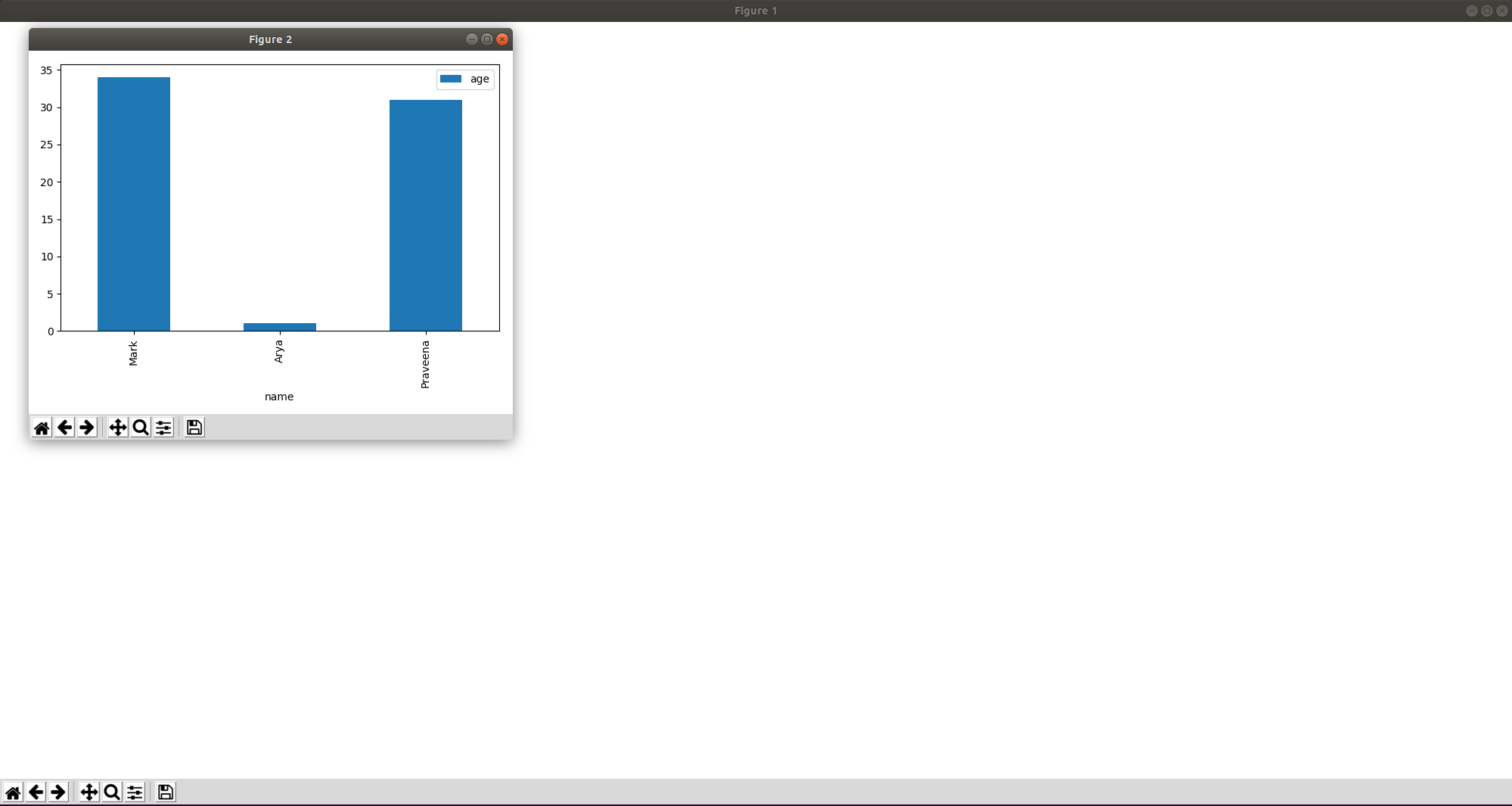# Python: Pandas - DataFrame plotting ignoring figure

In my continued use of matplotlib I wanted to change the size of the chart I was plotting and struggled a bit to start with. We’ll use the same DataFrame as before:

``````df = pd.DataFrame({
"name": ["Mark", "Arya", "Praveena"],
"age": [34, 1, 31]
})
df``````

In my last blog post I showed how we can create a bar chart by executing the following code:

``````df.plot.bar(x="name")
plt.tight_layout()
plt.show()
plt.close()``````

But how do we make it bigger? We can control this by using the `figure` function.

I gave this a try:

``````plt.figure(figsize=(20,10))
df.plot.bar(x="name")
plt.tight_layout()
plt.show()
plt.close()``````

If we run that we’ll see this output:Hmmmm…​we now have two figures, and the bigger one is completely blank! That’s not quite what we expected.

I came across a really thorough StackOverflow post which explained a variety of ways to solve the problem. The first way is to specify the `figsize` parameter when we call the `bar` function:

``````df.plot.bar(x="name", figsize=(20,10))
plt.tight_layout()
plt.show()
plt.close()``````

If we execute that code we’ll now have our big chart:There are another couple of ways we can achieve this as well. The `plot` function takes in a `ax` parameter, to which we can pass an existing Axes.

The `gca` function on our plot returns the current Axes instance or creates a new one:

``````plt.figure(figsize=(20,10))
df.plot.bar(x="name", ax=plt.gca())
plt.tight_layout()
plt.show()
plt.close()``````

Or rather than using the `gca` function on `plt`, we can capture the axes from the `figure` function and pass it in directly:

``````fig = plt.figure(figsize=(20,10))
df.plot.bar(x="name", ax=fig.gca())
plt.tight_layout()
plt.show()
plt.close()``````